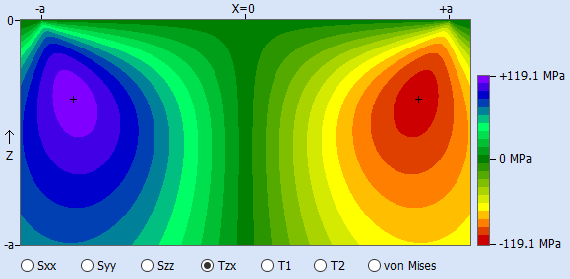+31(0)643 879 398 info(at)vinksda.nl
Select Page

Up to and including release 3.2.0 of HertzWin, all calculated results related to a static situation. The most important material stresses are then the maximum shear stress and the Von Mises equivalent stress. Most commonly used to determine whether a material is overloaded or not is the Von Mises equivalent stress. The maximum shear stress can be used for the Tresca criterion. Both criteria, Von Mises and Tresca, are usually close to each other in value and also in location: on the centerline of the contact (without tangential load), below the contact surface.

## Dynamic

The evaluation of stress is different in a dynamic situation, such as a rolling contact in bearings. Looking at the point where the maximum von Mises stress (or shear stress) occurs, the stress value in a rolling contact at this point in the material varies between zero and the maximum value.
Other points in the material undergo different stress changes. An important stress for fatigue in a rolling contact is shown in the picture below. It shows the orthogonal shear stress in the X-direction in the XZ-plan (figure made with HertzWin).Yet another stress that is seen as important in fatigue life is octahedral shear stress. However, opinions on which one can be used best, are divided. See also T.A. Harris, M.N. Kotzalas, Essential Concepts of Bearing Technology.

## Stress Variation

The maxima of the orthogonal shear stresses are located near the edge of the contact, some distance below the contact surface. The maximum value of this orthogonal shear stress is always slightly lower than the maximum shear stress on the centerline. But it does vary from maximum positive to a maximum negative value. For fatigue this is a more important stress change than between zero and maximum value.

Note: the variation of the stress in the material between a negative and a positive value only occurs in the direction of rolling. Thus, when the contact rolls in the X direction, so to speak, the orthogonal shear stress Tzx varies between the negative and positive maxima. The orthogonal shear stress Tzy then varies between zero and the positive maximum on one side of the contact and between zero and the negative maximum on the other side of the contact.

## Calculation

The value of the orthogonal shear stress depends on the shape of the contact surface: circular, elliptical or a line (rectangle). The stresses are simply calculated according to the equations given in K.L. Johnson, Contact Mechanics. As of release 3.3.0, the orthogonal shear stress is calculated in HertzWin.
The octahedral shear stress τoct is easy to calculate from the Von Mises stress: τoct = (vonMises*√2)/3.

Two other interesting articles on rolling contact fatigue (RCF) in a rolling contact are: